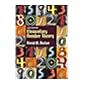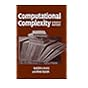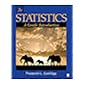Normal view

# Statistics : a gentle introduction / Frederick L. Coolidge.

Material type:TextPublication details: Thousand Oaks, Calif. : SAGE Publications, c2013.Edition: 3rd edDescription: xxiv, 458 p. : ill. ; 24 cmISBN:
• 9781412991711 (pbk.)
• 1412991714 (pbk.)
Subject(s): LOC classification:
• QA276.12  .C67 2013
Contents:
1. A gentle introduction -- 2. Descriptive statistics: understanding distribution of numbers -- 3. Statistical parameters: measures of central tendency and variation -- 4. Standard scores, the z distribution, and hypothesis testing -- 5. Inferential statistics: the controlled experiment, hypothesis testing, and the z distribution -- 6. An introduction to correlation and regression -- 7. The t test for independent groups -- 8. The t test for dependent groups -- 9. Analysis of variance (ANOVA): one-factor completely randomized design -- 10. After a significant analysis of variance: multiple comparison tests -- 11. Analysis of variance (ANOVA): one-factor repeated-measures design -- 12. Factorial ANOVA: two-factor completely randomized design -- 13. Post hoc analysis of factorial ANOVA -- 14. Factorial ANOVA: additional designs -- 15. Nonparametric statistics: the chi-square test -- 16. Other statistical parameters and tests -- Appendix A: z distribution -- Appendix B: t distribution -- Appendix C: Spearman's correlation -- Appendix D: The chi-square distribution -- Appendix E: F distribution -- Appendix F: Tukey's table.
Item type:Books List(s) this item appears in: Statistics
Holdings
Current library Collection Call number Vol info Status Date due Barcode
Judith Thomas Library General Stacks BKS QA 276.12 .C67 2013 (Browse shelf(Opens below)) AUA014553 Available AUA014553
##### Browsing Judith Thomas Library shelves, Shelving location: General Stacks Close shelf browser (Hides shelf browser)QA 241 .B83 2007 Elementary number theory / QA 267.7 .A76 2009 Computational complexity : QA 267.7 .A76 2009 Computational complexity : QA 276.12 .C67 2013 Statistics : QA 276.2 .L83 2002 Quantitative Techniques / QA 303 .S94 1983 Calculus with analytic geometry / QA 303.2 .H64 2007 Student's solutions manual to accompany calculus for business, economics, and the social and life sciences, brief ninth edition /

Includes bibliographical references (p. 449-450) and index.

1. A gentle introduction -- 2. Descriptive statistics: understanding distribution of numbers -- 3. Statistical parameters: measures of central tendency and variation -- 4. Standard scores, the z distribution, and hypothesis testing -- 5. Inferential statistics: the controlled experiment, hypothesis testing, and the z distribution -- 6. An introduction to correlation and regression -- 7. The t test for independent groups -- 8. The t test for dependent groups -- 9. Analysis of variance (ANOVA): one-factor completely randomized design -- 10. After a significant analysis of variance: multiple comparison tests -- 11. Analysis of variance (ANOVA): one-factor repeated-measures design -- 12. Factorial ANOVA: two-factor completely randomized design -- 13. Post hoc analysis of factorial ANOVA -- 14. Factorial ANOVA: additional designs -- 15. Nonparametric statistics: the chi-square test -- 16. Other statistical parameters and tests -- Appendix A: z distribution -- Appendix B: t distribution -- Appendix C: Spearman's correlation -- Appendix D: The chi-square distribution -- Appendix E: F distribution -- Appendix F: Tukey's table.